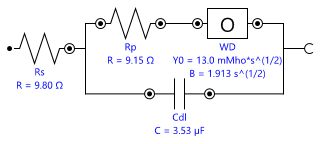You have been redirected to your local version of the requested page

The mass transport characteristics of the diffusion controlled oxidation and reduction of the ferri/ferro cyanide couple was studied using the Autolab RDE with a low noise liquid Hg contact.

Linear sweep voltammetry (LSV) and electrochemical impedance spectroscopy (EIS) experiments were performed on a 3 mm diameter platinum disk immersed in an electrolyte containing 0.05 M potassium ferrocyanide (K4[Fe(CN)6]) and 0.05 M potassium ferricyanide (K3[Fe(CN)6]) in 0.2 M NaOH supporting electrolyte. The electrode was polished to 3 μm finish before the start of the experiment. A large area platinum counter electrode and an Ag/AgCl (KCl saturated) reference electrode were used for the measurements.

For the EIS measurements, a 50 nF capacitor was put in parallel with the reference electrode to compensate for the phase shift introduced by the slow response of the reference electrode at high frequencies.

For the LSV experiments, the potential was swept between -0.5 V and 0.5 V vs. open circuit potential (OCP). A scan rate of 0.1 V/s was used for the measurements. The EIS measurements were conducted at OCP with 10 mV potential perturbation. A frequency range from 100 kHz to 0.1 Hz was used.

Measurements were performed using a Metrohm Autolab PGSTAT302N equipped with a FRA32M module. The LSV and EIS measurements were performed using the Autolab NOVA software. The rotation speed of the RDE was controlled directly from the software. The rate was varied from 100 rpm to 3200 rpm.

The LSV results for the various rotation rates are shown in Figure 1. The oxidation and reduction limiting currents increased with the increase in rotation speed.

Figure 1. Overlay of the LSV curves recorded at different rotation rates using the Autolab RDE. Light blue: 100 RPM; red: 200 RPM; yellow: 400 RPM; green: 800 RPM; purple: 1600 RPM; dark blue: 3200 RPM.

In Figure 2, the anodic (A) and cathodic (B) limiting currents (absolute values) are plotted as a function of the square root of rotation speed.

Figure 2. The Levich plots obtained by plotting the absolute values of the limiting currents versus the square root of the angular frequency. A - blue dots: anodic limiting currents. B - red triangles: cathodic limiting currents.

The data points fall exactly on a straight line as predicted by Levich theory, Equation 1.

Where:
(cm2) is the area of the electrode
n  is the number of electrons involved in the redox reaction
F (96485 C mol-1) is Faraday’s constant
C (mol cm-3) is the bulk concentration of the electroactive species
D (cm2 s-1) is the diffusion coefficient
𝜈 (cm2 s-1) is the kinematic viscosity of the solution
𝜔 (rad s-1)is the angular rotation rate

The Bode plots for the EIS measurements are shown in Figure 3.

Figure 3. Bode plot (phase shift in blue data and module of the impedance in red data) for each rotation rate. Triangles: 100 RPM; circles: 200 RPM; squares: 400 RPM; flags: 800 RPM; crosses: 1600 RPM; dotted lines: 3200 RPM. The solid lines are the fit results.

The Nyquist plots of the EIS measurements are shown in Figure 4.

Figure 4. Nyquist plot for each rotation rate. Data are in points and the fit results are in solid lines. Light blue: 100 RPM; red: 200 RPM; yellow: 400 RPM; green: 800 RPM; purple: 1600 RPM; dark blue: 3200 RPM.Figure 5. The equivalent circuit used to fit the data in Figure 3 and Figure 4.

In Figure 5, the equivalent circuit used to fit the EIS data is shown.

At high frequencies, the impedance is independent of the rotation rate of the RDE. The semicircle corresponds to the fast oxidation and reduction kinetics, fitted with the Rs(RpCdl) part of the equivalent circuit.

At low frequencies, the impedance decreases with the increasing of the rotation rate, resulting in a finite-length diffusion which can be fit with the Warburg – shot circuit terminus element, WD in the equivalent circuit of Figure 5.